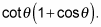##### Trigonometry Workbook For DummiesWhen a trigonometric expression is a fraction with a binomial in its denominator, always consider multiplying by the conjugate before you do anything else. Most of the time, this technique allows you to simplify.

For example, follow the steps to rewrite this expression without a fraction: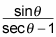1. Multiply the numerator and denominator by the conjugate of the denominator.

The conjugate of a + b is ab, and vice versa. So you have to multiply by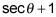on the top and bottom of the fraction. This step gives you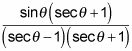2. FOIL the conjugates.

Remember that when you FOIL, you multiply the first, outside, inside, and last terms together.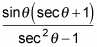3. Change any identities to their simpler forms.

Using the identity on the bottom, you get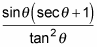4. Change every trig function to sines and cosines.

Here it gets more complex: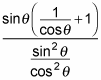5. Change the big division bar to a division sign, and then invert the fraction so you can multiply instead.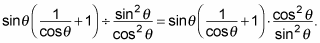6. Cancel what you can from the expression.

The sine on the top cancels one of the sines on the bottom, leaving you with the following equation: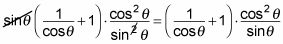7. Distribute and watch what happens!

Through cancellations, you go from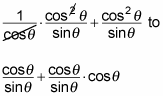This expression finally simplifies to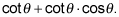And if you're asked to take it even a step further, you can factor to get